# Calculators

 < Day Day Up >

Word processing is perhaps one of the most common activities on any computer. Another very common activity is mathematical calculations of one sort or another. The need for number crunching is a fact of life. We all have occasion to need the accurate processing of numbers. Even if you are not an accountant, you will still need to balance checkbooks, compute interest rates on your credit card, and perform dozens of other functions with a calculator. You have probably used the Windows Calculator program before. This program is found by selecting Start, choosing Programs, going to Accessories, and selecting the Calculator, shown in Figure 5.10.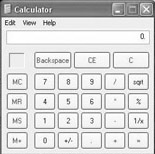Figure 5.10: The Windows Calculator.

The Windows Calculator is well suited for basic arithmetic operations. The mathematics involved in bookkeeping, computing a loan interest rate, or working with your budget, for example, can readily be accomplished with this calculator. However, if everyday math is not suited to your task, you can select View from the drop-down menu and switch to a scientific calculator, shown in Figure 5.11.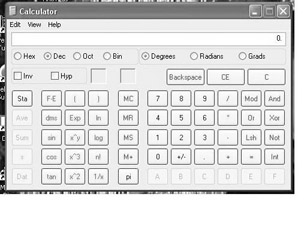Figure 5.11: The Windows scientific calculator.

The scientific calculator enables you to work with various exponents, roots, scientific notation, and more. This calculator is appropriate for the mathematics associated with general chemistry courses (either in secondary school or for freshmen college courses) as well as algebra-based physics courses. You have probably already guessed that Linux has an answer to your calculator needs. If you look under Accessories, you will find a scientific calculator, shown in Figure 5.12. An important side note should be made at this juncture. You have probably already noticed that Linux has applications that are comparable to all of the applications you have found in Windows. You should also notice that very often they are even found in the same place! Notice how the calculators in both operating systems are located under Accessories.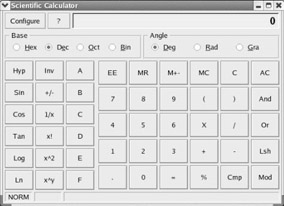Figure 5.12: The Linux Scientific Calculator.

By examining the Windows scientific calculator and the Linux Scientific Calculator, or simply by looking closely at Figures 5.11 and 5.12, you should note a number of similarities. Both have the option to select hexadecimal, decimal, octal, or binary number systems. Both also give you the option of having angles represented in one of three different ways. This option is of particular interest if you intend to use your calculator for trigonometry. Both give you buttons for basic trigonometry functions (sine, cosine, tangent), raising a number to a certain power, computing the logarithm or the natural logarithm of a number, and more. In fact, you may be tempted to claim that the two have identical functionality and only slightly differing layouts. If you made such a claim, you would be almost correct, but only almost. If you notice the Configure button that is prominent in the upper-left corner of the Linux calculator and conspicuously absent from the Windows Calculator, you will find the difference between the two. This button may seem a small difference, but it offers a great deal of flexibility. Clicking it takes you to the Configuration screen, shown in Figure 5.13.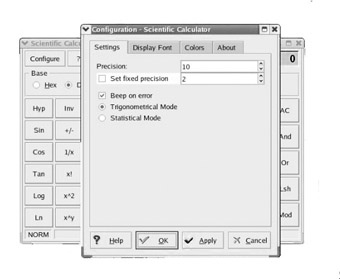Figure 5.13: The Calculator Configuration screen.

There are four tabs on this screen, each enabling you to alter a different aspect of the Linux Scientific Calculator. The middle two options merely alter the appearance of your calculator. You may select any font or color combination that you find appealing. The first tab, Settings, is the one that enables you to change how the calculator will behave. Here you can alter the precision with which mathematical calculations are done. This is of critical importance to students in science classes, where the precision of a calculation is of paramount importance to successfully solve a given. You also can customize the calculator for either trigonometric or statistical use. Finally, you can elect to have the calculator beep if an error occurs.

As you can see, Linux offers all the calculator functionality you have grown accustomed to in Windows. In fact, using the Scientific Calculator that comes with KDE, you have some functionality not present in the Windows scientific calculator. We have found again that in Linux, not only can you perform all the tasks you are used to doing in Windows, but you may even be able to perform some tasks you could not do in Windows. Of course, some readers may be wondering what some of the mathematical functions listed do. You might not be familiar with natural logarithms or measuring an angle in radians. If this is the case, rest comfortably knowing that Linux not only is ready to meet your current mathematical needs, but is capable of doing even more!

 < Day Day Up >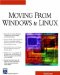Moving From Windows To Linux (Charles River Media Networking/Security)
ISBN: 1584502800
EAN: 2147483647
Year: 2004
Pages: 247
Authors: Chuck Easttom